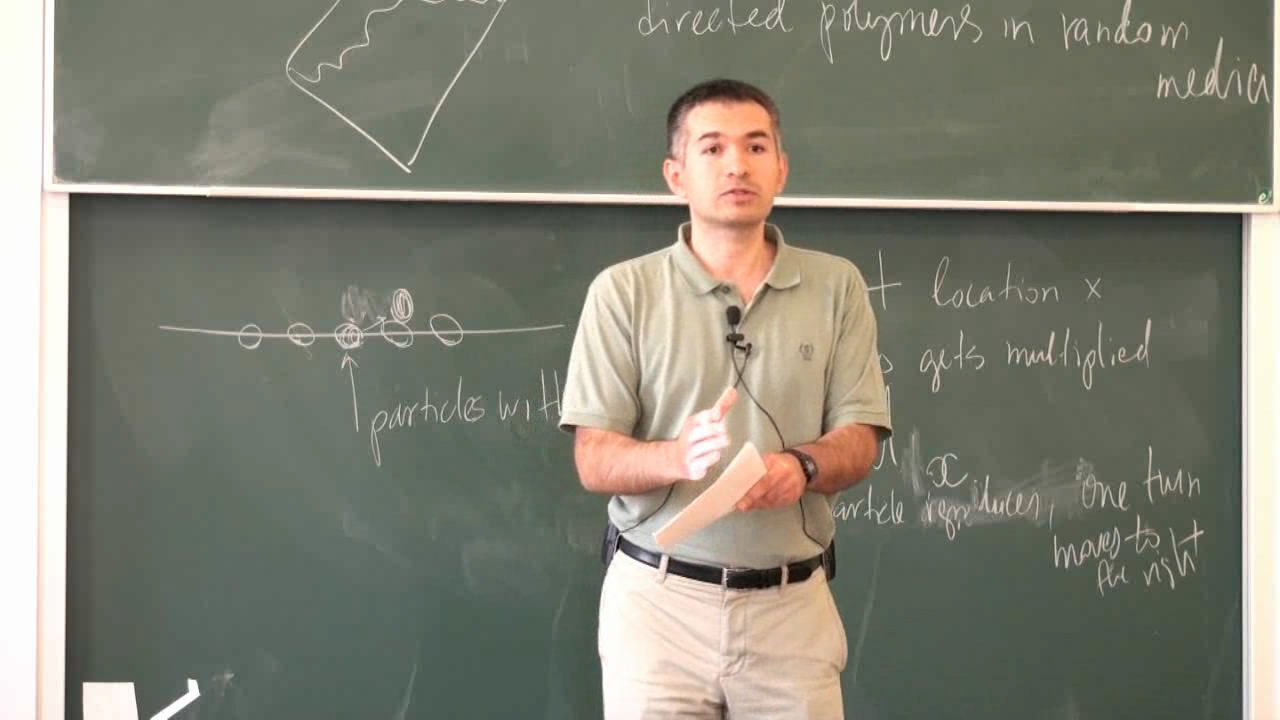# Integrable models of random growth and branching graphs

Курс Хит
Предмет:
Лектор:The goal of the mini-course is to explain how representation theory helps to discover and analyze integrable structures in probability. The relevant representation theoretic constructions involve representations and characters of symmetric and unitary groups and their infinite-dimensional analogs, Schur and Macdonald symmetric polynomials, and Whittaker eigenfunctions of the quantum Toda lattice. The probabilistic counterparts are random growth models in one and two space dimensions, last passage percolation and directed polymers, solutions of the stochastic heat equation and Kardar-Parisi-Zhang equation and associated quantum many-body systems.

Курс прочитан в рамках St. Petersburg School in Probability and Statistical Physics.

Источник информации

8